Subsections

# Density operator formalism

When dealing with systems with many qubits, it is often inconvenient to use state vectors to describe the system as the required computational power grows exponentially. In the case of non-interacting subsystems the problem can be solved by using the density operator formalism. In this formalism the quantum state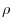is represented by a statistical mixture of state vectors where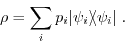Here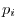denotes the probability of finding the system in the state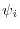[Breuer, 2002].

## Structure

libquantum provides an interface for density operators:

 quantum_density_op {    int num; /* total number of state vectors */    float *prob; /* probabilities of the state vectors */    quantum_reg *reg; /* state vectors */ };

## quantum_new_density_op

 extern quantum_density_op quantum_new_density_op(int num, float *prob, quantum_reg *reg);

Density operators can be created from already existing quantum registers. The parameter num denotes of how many quantum registers the density operator shall consist. Therefore both prob and reg have to be arrays of the length num. All quantum registers in reg will be destroyed by the function.

## quantum_qureg2density_op

 extern quantum_density_op quantum_qureg2density_op(quantum_reg *reg);

It is possible to generate a density operator from a single quantum register. Note that the quantum register passed to the function will be destroyed.

## quantum_delete_density_op

 extern void quantum_delete_density_op(quantum_density_op *rho);

Destroys the given density operator and frees its memory.

## quantum_print_density_matrix

 extern void quantum_print_density_matrix(quantum_density_op *rho);

This function prints the matrix that is associated with the density operator to the standard output stream. The matrix elements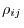are given by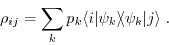## quantum_reduced_density_op

 extern void quantum_reduced_density_op(int pos, quantum_density_op *rho);

In order to perform the transition from a pure quantum state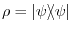to a mixed state, the reduced density operator can be calculated. Technically, this is done by computing the partial trace of the subsystem denoted by the qubit pos.

## quantum_purity

 extern float quantum_purity(quantum_density_op *rho);

Returns the purity of the density operator rho, which is defined as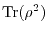.

## quantum_density_operation

 #define quantum_density_operation(function, rho, ...)

This preprocessor macro provides a wrapper around the quantum operations provided by libquantum. The operation is given by function, the density operator by rho, followed by the parameters to function without the quantum register. It is possible to use this wrapper for arbitrary functions if the last parameter of the function is the quantum register.

The macro currently does not work with

while the latter should not be used within the density operator formalism anyway. To use these functions you can create a loop over all quantum registers of the density operator. For example:

  1 2 3 4 5  `int i; quantum_density_op rho; for(i=0; i